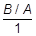# Activities

•##### Download

•• ##### Author6-8

• ##### Device
•TI-Nspire™ CX/CX II
•TI-Nspire™ CX CAS/CX II CAS
• TI-Nspire™ Apps for iPad®
• ##### Software

TI-Nspire™
TI-Nspire™ CAS

• ##### Report an Issue

Proportional Relationships

#### Activity Overview

This activity allows students to reason about ratio tables, which helps their understanding of what a ratio describes in a context and what quantities in equivalent ratios have in common.

#### About the Lesson

This TI-Nspire™ lesson focuses on the relationship between the unit rate or slope of a line and the equation. The graph of a proportional relationship passes through the origin, and as a consequence, the graph is completely determined by one point, often the point representing the unit rate, (1,).

Prior to working on this lesson, students should understand:

• the members of any set of equivalent ratios have the same unit rate;
• the unit rate is a measure of the steepness of the slope;
• the constant of proportionality, k, is the unit rate or the slope of a line through the origin.

This is Lesson 11 out of 15 in the Building Concepts in Mathematics: Ratios and Proportional Relationships Series